## MP Board Class 11th Physics Important Questions Chapter 14 Oscillations

Oscillations Class 11 Important Questions Very Short Answer Type

Question 1.
Define simple harmonic motion with example.
Answer:
When a body moves to and fro about a point in a straight line such that its acceleration is always directly proportional to the displacement and directed towards the mean position, then its motion is called simple harmonic motion.
Example: Motion of a body suspended by a spring, motion of simple pendulum.

Question 2.
What is oscillatory motion?
Answer:
When a body moves periodically to and fro or back and forth about a definite point, then its motion is called oscillatory motion.
Motion of simple pendulum, vibration of tuning fork etc. are the examples of oscillatory motion.

Question 3.
For what type of motion of a body acceleration is directly proportional to displacement?
Answer:
It is simple harmonic motion.

Question 4.
When the velocity and acceleration will be maximum in S.H.M.?
Answer:
The velocity is maximum at the mean position and acceleration is maximum at the extreme position.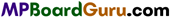Question 5.
Write the formula for kinetic energy and potential energy for a body executing S.H. M.?
Answer:
Kinetic Energy = $$\frac{1}{2} m \omega^{2}\left(a^{2}-y^{2}\right)$$
Potential Energy = $$\frac{1}{2} m \omega^{2} y^{2}$$
Where m – particle of mass,
ω – angular frequency,
a – amplitude and
y = displace-ment of the particle form its mean position.

Question 6.
At which position kinetic energy and potential energy is zero for a body executing S.H.M.?
Answer:
At maximum displacement position (y = ±a) kinetic energy is zero and at mean position (y = 0) potential energy is zero.

Question 7.
Define simple pendulum.
Answer:
When a heavy point mass is suspended by a weightless, inextensible and perfectly flexible string by a rigid support, then it is called simple pendulum.

Question 8.
Which of the following relationships between acceleration ‘a’ and the dis-placement x: of a particle involve simple harmonic motion ? (NCERT)
(a) a = 0.7x,
(b) a = 200x2,
(c) a = -10x2
(d) a = 100x3.
Answer:
In a S.H.M. the relation between acceleration and displacement is
a=-ω2x
(a) a = 0.7 x
Comparing with eqn. (1),
2 = 0.7
or’
ω = $$\sqrt{-0 \cdot 7}$$
∵ Value of a is not real therefore the motion is not S.H.M.

(b) a = -200x2
This motion is not a S.H.M.

(c) a = -10x
Comparing-with eqn. (1),
ω2 =10
or
ω = $$\sqrt{10}$$
∵ ω has a real value therefore, the relation shows a S.H.M.

(d) a = 100 x3
This motion is not a S.H.M.

Question 9.
The length of a spring is increased, how the time-period be affected?
Answer:
The time-period will increase.

Question 10.
On which conservation law S.H.M. is based?
Answer:
S.H.M. is based on law of conservation of energy.

Question 11.
What is second pendulum? What is its length?
Answer:
The pendulum whose periodic time is two seconds is known as second pendulum. The length of second pendulum is about 100 cm.

Question 12.
Write the characteristics of S.H.M.
Answer:
Characteristics of S.H.M.:

• The motion is periodic.
• The particle moves to and fro about a point in a straight line.
• The acceleration is directly proportional to the displacement.
• The direction of acceleration is always towards the mean position.

Question 13.
Define time-period, frequency and amplitude.
Answer:
Time period: The time taken by an oscillating body to complete one oscillation is called time-period.
Frequency: The number of vibrations or oscillations in one second is called frequency.
Amplitude: The maximum displacement of a particle about its mean position is called amplitude. It is denoted by a or A.Question 14.
What is the relation between S.H.M. and Circular motion?
Answer:
When any particle is performing uniform motion on the circumference of a circle, then the perpendicular dropped on the diameter of the circle from any point of rotation execute S.H.M. along the diameter of circle.

Question 15.
Define spring constant and write its S.I. unit and dimensional formula.
Answer:
Spring constant: The force required to elongate or compress a spring through unit length is called spring constant.
Unit: SI unit of spring constant is Nm1.
Dimensional formula : Dimensional formula is [ML°T-2].

Question 16.
Write the formula for the time-period of a body suspended by a spring.
Answer:
Where, T= Time-period, m = Mass suspended and k = Force constant.

Question 17.
What is meant by the effective length of a simple pendulum?
Answer:
The length of pendulum from the point of suspension to the centre of bob is called effective length.

Question 18.
Why the length of simple pendulum is measured up to the centre of the bob?
Answer:
The centre of gravity point of spherical bob lies at its centre. The concept of heavy point mass is assumed that the weight of the body acts at the C.G. point. Therefore the effective length is measured up to centre of the bob.

Question 19.
How time period of a simple pendulum change with effective length of a simple pendulum.
Answer:
We know $$T=2 \pi \sqrt{\frac{l}{g}}$$
or
T ∝ $$\sqrt{l}$$
When effective length is increased, time period also get increased.

Question 20.
Can the pendulum clock be used in a satellite?
Answer:
No, because g = 0 inside satellite, therefore T = ∞.
So, in place of pendulum clock, spring clock can be used inside it.Question 21.
The pendulum clock cannot be used in artificial satellite. Why?
Answer:
In an artificial satellite, the bodies are in the state of weightlessness. Hence, g becomes zero. Thus, the time-period becomes infinite.Therefore, it cannot be used in the satellite.

Question 22.
A boy is swinging, sitting on a swing. If another boy sits beside him, what will happen to its time-period?
Answer:
The time period is independent of mass, hence there will be no effect.

Question 23.
A boy is swinging, standing on a swing. If he sits on it, what happen to the time-period?
Answer:
When the boy will sit on the swing, his C.G. point will shift downwards. Hence, the effective length will increase therefore the time-period will also increase.

Question 24.
What is the reason that the frequency of oscillation clocks depend on the rise and fall of mercury level in the thermometer?
Answer:
If the mercury level increases, it shows that temperature has increased and the decreases in mercury level shows a decrease in temperature. Due to increase in temperature, the length of pendulum increases and $$T \propto \sqrt{l}$$ so time period also increases. Hence clock runs slow, i.e., the frequency of oscillation decreases. But with decrease in temperature, length decreases and so time-period decreases. Hence clock runs fast i.e., frequency of oscillation increases.

Question 25.
The pendulum clock becomes fast when it is taken to pole from equator. Why?
Answer:
We have, T = $$2 \pi \sqrt{\frac{l}{g}}$$
If l is constant ,then T ∝ $$\frac{1}{\sqrt{g}}$$
Hence, time-period is inversely proportional to the square root of acceleration due to gravity. At poles the value of g is greatest, hence the time-period will decrease at poles, hence the clock will be fast.Question 26.
Why pendulum clock does not show time at satellite?
Answer:
At satellite g = 0, therefore, i.e., time period of pendulum become infinity, therefore it does not show time of satellite.

Question 27.
What is damped oscillation?
Answer:
When the amplitude of an oscillating body decrease gradually by some external opposing force, then its oscillation is called damped oscillation.

Question 28.
Define forced vibration.
Answer:
When an oscillating body oscillates under the influence of some external force, then it oscillates with the frequency of external force, then its oscillation is called forced vibration.

Question 29.
What do you mean by forced oscillation?
Answer:
When the body oscillate under the influence of an external periodic force, with a constant amplitude and a frequency equal to the periodic force, the oscillation of the body is called forced oscillation.

Question 30.
Define resonance.
Answer:
When a body vibrates under the influence of a periodic force and its frequency becomes equal to that of periodic force then the amplitude of the body increases. This phenomenon is known as resonance.

Question 31.
Why marching troops are asked to break their steps while crossing the bridge?
Answer:
The footsteps of marching troops produces a periodic force on the bridge. If the frequency of foot-steps becomes equal to the natural frequency of bridge, the amplitude of bridge will increase and hence the bridge may break.Question 32.
What is the effect of forced oscillation on amplitude and frequency?
Answer:
Due to forced oscillation amplitude of oscillating body go on reducing and frequency become less than the natural frequency.

Question 33.
When forced oscillation become resonance oscillation?
Answer:
When the natural frequency of the body becomes equal to the frequency due to periodic force then forced oscillation is converted into resonance oscillation.

Question 34.
A simple pendulum is suspended in a lift, whose time-period is T. What will aeration ‘a’ (i) upward, (ii) downward.
Answer:
(i) When the lift accelerate with acceleration ‘a’ upward then the time-period will decrease
( T = $$2 \pi \sqrt{\frac{l}{g+a}}$$)
(ii) On acceleration with acceleration ‘a’ downward then its timeperiod will increase
( T = $$2 \pi \sqrt{\frac{l}{g-a}}$$ )

Question 35.
What will be the time-period of effective length infinity?
Answer:
84.6 minute.

Question 36.
What will be the time period of pendulum at the centre of earth? Why?
Answer:
At the centre of earth the time-period of pendulum will be infinity as ‘g’ is zero at the centre.

Question 37.
A spring of spring constant k is cut into three equal pieces. What will be the time period of each?
Answer:
The spring constant of each piece will be 3k.

Oscillations Class 11 Important Questions Short Answer Type

Question 1.
The bob of simple pendulum is a ball filled with water, if a small hole is made at the bottom of the ball, how will its time period change as water is drains out of it?
Answer:
When the ball is completely filled with water, its centre of gravity be at its geometric centre. So, it will oscillate with definite time period. But as the water drains out of it through the hole, the centre of gravity is displaced gradually downward. Hence the effective length also increases gradually. Therefore the time period of the pendulum also increases gradually. But when whole of the water is removed then its centre of gravity again shifted to its geometric centre and its time-period become same as was in initial position.

Question 2.
What is simple harmonic motion. Write its four characteristics.
Answer:
When a body moves to and fro about a point in a straight line such that its acceleration is always directly proportional to the displacement and directed towards the mean position, then its motion is called simple harmonic motion.
Example: Motion of a body suspended by a spring, motion of simple pendulum.
Characteristics of S.H.M.:

• The motion is periodic.
• The particle moves to and fro about a point in a straight line.
• The acceleration is directly proportional to the displacement.
• The direction of acceleration is always towards the mean position.

Question 3.
Write the relation between acceleration and displacement of a particle ex-ecuting1 S.H.M. Also deduce expression for time-period from it?
Answer:
Acceleration of the particle with displacement y is α = -ω2y
Where ω is angular velocity of particle here (-) sign indicate the direction of accel-eration only.
Hence, ω2 = $$\frac{\alpha}{y}$$ = $$\frac{\text { Acceleration }}{\text { Displacement }}$$
or
ω = $$\sqrt{\frac{\alpha}{y}}$$
But T =$$\frac{2 \pi}{\omega}=\frac{2 \pi}{\sqrt{\frac{\alpha}{y}}}=2 \pi \sqrt{\frac{y}{\alpha}}$$
T = $$2 \pi \sqrt{\frac{\text { Displacement }}{\text { Acceleration }}}$$Question 4.
Write the expression for displacement, velocity and acceleration of a particle executing S.H.M. and say :
(i) When the velocity of the particle will be maximum and zero.
(ii) When the acceleration of the particle will be maximum and zero.
Answer:
Displacement y = a sin ωt
Velocity ν = ω $$\sqrt{a^{2}-y^{2}}$$
Acceleration a = – ω2y
Where a is amplitude and ω is angular acceleration.

(i) Velocity is maximum at mean position (y = 0)
νmax = ω.a
Velocity is zero at maximum displacement position ν = 0 , when y = ±a.

(ii) Acceleration is maximum when displacement is maximum (y = ±a)
α = ± ω2 a, when y = ±a
Acceleration is zero at mean position α = 0, when y = 0.

Question 5.
What is meant by simple pendulum? When the bob is displaced from its mean position it starts oscillations. Why?
Answer:
If a heavy point mass be suspended by a weightless, perfectly flexible and inextensible string from a rigid support and an arrangement is made for its frictionless oscillations, then this arrangement is called simple pendulum. As shown in fig. when bob is displaced from its mean position A to a position B, then the bob raised up to height ‘h\ so that its potential energy increases.

Now, if the bob is released, then its centre of gravity falls down to obtain the stable equilibrium and when it reaches to the mean position its potential energy is converted into kinetic energy. At points, it will not stay, but due to inertia moves forward and hence the bob starts to execute simple harmonic motion to and fro about its mean position.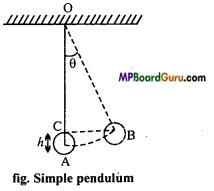Question 6.
Write down the formula of time-period of simple pendulum and state the factors on which it does depend.
Answer:
The time-period of simple pendulum is given by :
T = 2π $$\sqrt{\frac{l}{g}}$$
From the above formula, it is clear that the time period of simple pendulum depends on its effective length ‘l’ and acceleration due to gravity ‘g’.
(i) Dependence on length f: The time period of simple pendulum is directly proportional to the square root of effective length of pendulum, i.e., T α $$\sqrt{l}$$
Example: When a boy swinging on a swing stand up suddenly, then his centre of gravity rises up and so the effective length of the swing reduces which results an increase in time period.

(ii) Dependence on acceleration due to gravity ‘g’: The time-period T of simple pendulum is inversely proportional to the square root of acceleration due to gravity at that place i.e., T α $$\frac{1}{\sqrt{g}}$$
Example: When a pendulum is taken up to hills or down in a mine get slow because time-period T increases due to decrease in the value of ‘g’.

Question 7.
Write down the laws of simple pendulum. Give practical application of each law.
Answer:
The laws of simple pendulum and their practical applications are given below :
(i) Law of length: If the value of ‘g’ remains constant, then the time period of simple pendulum is directly proportional to the square root of effecti ve length of simple pendulum.
i-e., T α $$\sqrt{l}$$
This law is used to repair the pendulum clocks when they get slow or becomes fast while taken up to hills or down in a mine.

(ii) Law of acceleration due to gravity : If the effective length of simple pendulum remains constant, then the time period T of the simple pendulum is inversely proportional to the acceleration due to gravity, i.e., T α $$\frac{1}{\sqrt{g}}$$
Due to this reason the pendulum clock get slow while taken up to hills or down in mines.

(iii) Law of mass: The time period of simple pendulum does not depend upon the mass of bob or that of thread.
Hence, either bob is heavy or lighter, if the value of 7’ and ‘g’ are constant, then there is no effect in its time-period. .

(iv) Law of isochronism: If the amplitude of oscillations of simple pendulum are very small, then the time-period of pendulum does not depend upon the amplitude of oscillations.
Because of this, the angular displacement of the bob is kept small in the experiment of simple pendulum.

Question 8.
Establish the relation between time-period and frequency.
Answer:
Let the time-period of a particle is T and its frequency is υ.
∴ The particle complete one vibration in T sec.
Hence, in 1 sec the number of vibration, υ = $$\frac{1}{T}$$
or
υT = 1.

Question 9.
Derive an expression for the displacement of a particle executing S.H.M.
Or
Find an expression for the displacement of S.H.M.
Answer:
Let XYX’Y’ is a circle whose centre is O and radius a. A particle is moving with uniform angular speed ω on the circle.
Let at time t = 0, the particle is at X and in time t, it is at P. A perpendicular PN is drawn on YOY’ from P and N is foot of the perpendicular.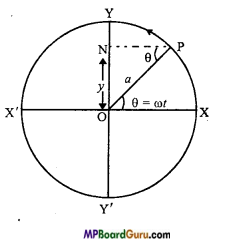The particle takes t second to subtend an ∠POX = θ.
∴ ω = $$\frac{\theta}{t}$$
or
θ = ωt
Let the displacement ON = y
∴ In ∆ NPO, sin NPO = $$\frac{O N}{O P}$$
But, ∠NPO = ∠POX = θ = ωt
∴ sin ωt = $$\frac{y}{a}$$
or
y = a sin ωt
This is the required equation for the displacement of S.H.M.Question 10.
Derive the expression for the velocity of a particle executing S.H.M.
Or
Derive an expression for velocity of a particle executing S.H.M. When is the velocity maximum and minimum?
Answer:
Expression for velocity: Let XYX’Y’ is a circle of centre O and radius a. A particle is moving on the circle with angular velocity ω.
Let at t = 0, the particle is at X and after t sec, it is at P.
Let at point P, the velocity of the particle is ν along the tangent at P.

Now, velocity v is resolved into two parts :
(i) ν sin θ, parallel to PN and
(ii) ν cos θ, perpendicular to PN.
Since,ν cos θ is parallel to the direction of motion of foot of the perpendicular N.
∴ Velocity of N, υ = ν cosθ
υ = ν cos ωt.
or
υ = ν $$\sqrt{1-\sin ^{2} \omega t}$$
( ∴ sin2 ωt + cos2 = 1)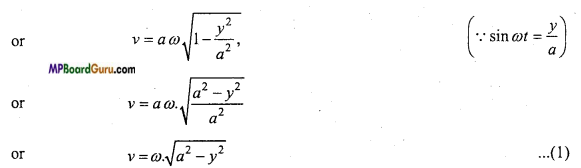This is the required expression.

Case 1. Ify = 0, then from eqn. (1), we get
ν =ω $$\sqrt{a^{2}-0}$$ = ωa
∴ Velocity will be maximum at mean position.
Case 2. If y = a, then from eqn. (1), we get
ν =ω $$\sqrt{a^{2}-a^{2}}=$$ = 0
∴ Velocity will be minimum at the extreme position.

Question 11.
Derive the expression for the acceleration of a particle executing S.H.M. Find where the acceleration is maximum and minimum.
Answer:
We have by displacement equation,
y = a sin(ωt + Φ) …(1)
If the acceleration is α, then we have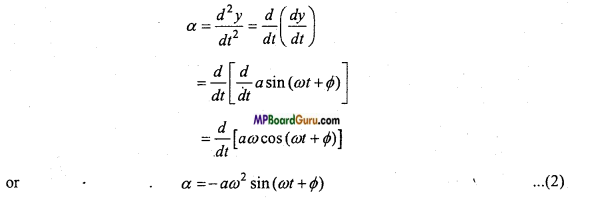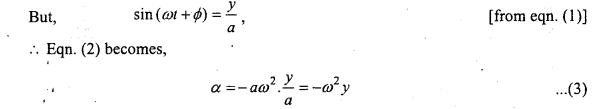This is the required expression.
Case 1. If y = 0, then by eqn. (3),
α =0
i.e., at the mean position the acceleration will be zero i.e., minimum.
Case 2. If y = a, then by eqn. (3),
α = – ω2a
i.e., at extreme position the acceleration is maximum.

Question 12.
Derive the expression for the time>period and frequency of a particle ex-ecuting S.H.M.
Answer:
Now, we have the magnitude of acceleration is given by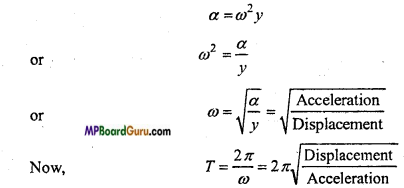Again, we know that frequency,
υ = $$\frac{1}{T}$$
∴ υ = $$\frac{1}{2 \pi} \sqrt{\frac{\text { Acceleration }}{\text { Displacement }}}$$

Question 13.
Mass m is suspended by an ideal spring. It oscillates up and down. If the force constant of spring Is k, then prove that the time-period,
T =2 π $$\sqrt{\frac{m}{k}}$$
or
A mass is suspended by a spring. It Is pulled downwards and then left. Prove that it executes S.H.M. Find the expression for time-period.
Answer:
Let a spring is suspended by a rigid support and mass ni is suspended at its lower end.
It is displaced downwards at a distance y and left.
The restoring force F = -ky …( 1)
Where, k is force constant.
Also, by Newton’s second law of motion,
F=mα …………. (2)
Where, α is acceleration.
∴ From eqns. (1)and (2), we get
mα =-ky ……….. (3)
or
α =$$-\frac{k}{m}$$ .y
Also, we have, for S.H.M.
α = – ω2 y ……..(4)
Since, the L.H.S. of eqns. (3) and (4) are equal, hence the R.H.S. must be equal.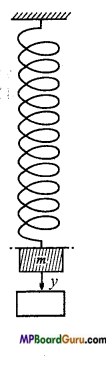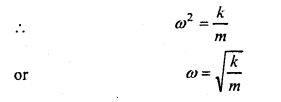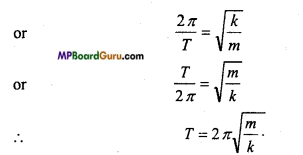Question 14.
Derive the expression for K.E. and P.E. of a particle executing S.H.M. and also prove that total energy of a particle remains constant.
Answer:
K.E. of the particle : We know that velocity of a particle executing S.H.M. is given by,
ν = ω$$\sqrt{a^{2}-y^{2}}$$

K.E.= $$\frac{1}{2}$$mv2
=$$\frac{1}{2}$$ m{ ω$$\sqrt{a^{2}-y^{2}}$$2
=$$\frac{1}{2}$$mω2(a2-y2) …(1)
P.E. of the particle: Now, the acceleration of a particle executing S.H.M. is given by α = -ω2y

Also, by Newton’s second law of motion,
Force = Mass x Acceleration
∴ F = mα
or
F = -mω2y
Now initially the displacement was zero and force was zero. The force was gradually increases and hence the displacement also increases to y.

∴Mean force =$$\frac{0+F}{2}$$ = mω2y
∵ Work W= Mean force x Displacement
∴ W = $$\frac{1}{2}$$ mω2y ×y
or
W = $$\frac{1}{2}$$ mω2y2

By equns.(1) and (2), we get
K.E +P.E = $$\frac{1}{2}$$ mω2 (a2-y2)+ $$\frac{1}{2}$$ mω2 y2
or
E = $$\frac{1}{2}$$ mω2a2 –$$\frac{1}{2}$$mω2y2 +$$\frac{1}{2}$$mω2y2
or
E = $$\frac{1}{2}$$mω2a2
As ω and a are constant, therefore the total energy remains constant.Question 15.
What is second’s pendulum? Calculate its effective length.
Answer:
Second’s pendulum: A simple pendulum whose time-period is 2 seconds, is called second’s pendulum.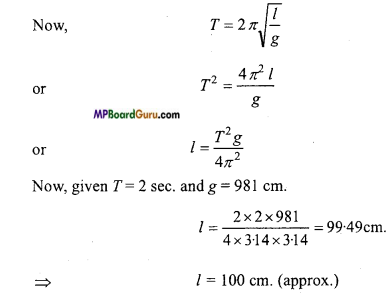Oscillations Class 11 Important Questions Long Answer Type

Question 1.
Deduce the expression for time-period of simple pendulum T = 2π $$\sqrt{\frac{l}{g}}$$
Answer:
Motion of a simple pendulum: Suppose that m is the mass of the bob and l is the effective length of a simple pendulum. S is a point of suspension and O is mean position of the bob. Suppose at any instant during oscillation, the bob is in the position P. Then displacement OP = y and ∠ OSP = θ. In this position of the bob, two forces act upon it:
(i) Tension T is the thread, in the direction PS upward along the thread and

(ii) The weight of the bob, mg, vertically downward. The weight mg can be resolved into two components :
(a) mg cos θ, in the direction SP downward along the thread.
(b) mg sin θ, perpendicular to the thread SP.
The component mg cos θ balances the tension Tin the thread. The component mg sin θ tends to bring the bob back to its mean position. This is known as restorting force on the bob. Thus, the restorting force acting on the bob is given as F= -mg sinθ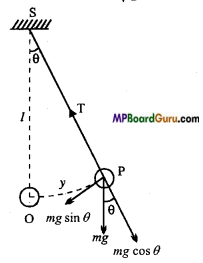Where negative sign shows that the direction of force is opposite to the direction which displacement increases, i.e., towards the mean position O.
1f the angular displacement of the bob be small, then sin θ = 0 (for example, if θ = 50, then sin θ= 0.0872 and θ=0.0873 radian). Thus,
F= -mgθ
But,
θ= $$\frac{\mathrm{Arc}}{\mathrm{Radius}}$$ = $$\frac{O P}{S P}$$ = $$\frac{y}{l}$$
F= -mg.$$\frac{y}{l}$$ …….. (1)

If α be the acceleration produced in the bob due to restoring force, then by Newton’s law of motion
F = mα ……. (2)
From eqns. (1) and (2), we get
mα = -mg $$\frac{y}{l}$$
or
α= –$$\frac{g}{l}$$.y …(3)

At a given place g is constant and for a given pendulum is constant.
Therefore,
α ∞ -y …….. (4)
Thus, the acceleration of the bob is directly proportional to its displacement. Hence, for small amplitude, the motion of a simple pendulum is simple harmonic motion. Its periodic time is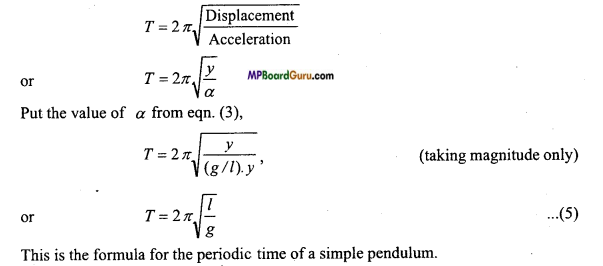Oscillations Class 11 Important Numerical Questions

Question 1.
The time-period of a particle executing S.H.M. is 2 sec. Calculate the time after t =0, that its amplitude behalf of the displacement.
Solution:
Given: T= 2 sec and y = $$\frac{a}{2}$$
∵ y = a sin ωt
Where ω =$$\frac{2 \pi}{T}$$
∴ y = a sin $$\frac{2 \pi}{T}$$ t ⇒ $$\frac{a}{2}=$$ = a sin $$\frac{2 \pi}{T}$$ t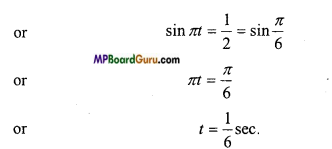Question 2.
The length of a simple pendulum ¡s 39.2 / π2 metre. If g = 9.8 ms-2, then calculate the time-period of simple pendulum.
Solution:
Given:l = $$\frac{39 \cdot 2}{\pi^{2}}$$, g = 9.8 ms-2
Formula: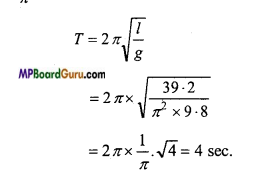Question 3.
The mass of a particle executing S.H.M. is 0.4 kg and amplitude and time period are 0.5 m and π/ 2 respectively. Calculate the velocity and ICE. of particle at a displacement 0.3 m.
Solution :
Given:m = 0.4kg , a = 0.5 m, T= π/2 sec, y = 0.3 m.
Npw we have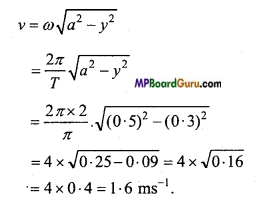Again,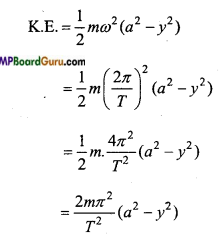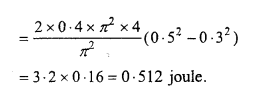Question 4.
A simple pendulum execute 60 oscillation per minute. Find its effective length ? (g = 981 cm/ s2)
Solution:
Given: Time-period T = $$\frac{\text { Time }}{\text { No.of oscillations }}$$ = \frac{1 \mathrm{~min}}{60} = 1 sec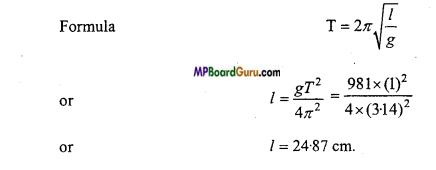Question 5.
The value of acceleration due to gravity on a planet is $$\frac{1}{4}$$ th of that of earth.
If the time-period of simple pendulum on earth is 2 sec, then find the time-period on the planet.
Solution:
g2= $$\frac{1}{4}$$ g1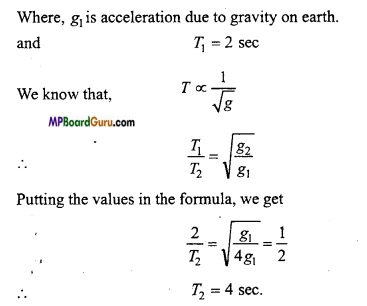Question 6.
What will be the time.period of a simple pendulum of length $$\frac{9 \cdot 8}{\pi^{2}}$$ m? Name such pendulum.
Solution:
Given: l= $$\frac{9 \cdot 8}{\pi^{2}}$$m g = 9.8 ms-2
Formula:
T =2π $$\sqrt{\frac{l}{g}}$$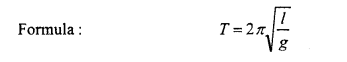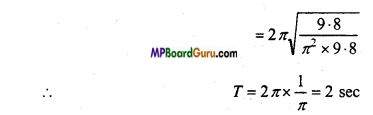Question 7.
Two pendulum of length 100 cm and 110*25 cm start oscillating at same time. After how much oscillation again they will oscillate at same time.
Solution:
Let the time-period of the 100 cm pendulum be T1
T1 = 2 π$$\sqrt{\frac{100}{g}}$$

and if T2is the time-period for 110.25 cm pendulum, then
T2 = 2 π$$\sqrt{\frac{110 \cdot 25}{g}}$$
T1 <T2
∴ Both the pendulum to oscillate at same time if the big pendulum perform n oscillaion and small pendulum, perform (n + 1) oscillation.
i.e.,(n+1)T1 = nT 2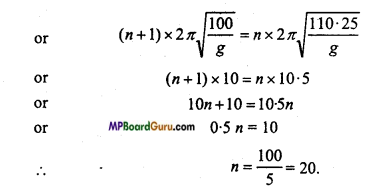i.e., Big pendulum after 20 oscillation or small pendulum after 21 oscillation will start oscillating together.Question 8.
Find the length of second pendulum at (i) Surface of earth (g = 9-8 m/s2), (ii) Surface of moon (g = 1.65 m/s2).
Solution:
Time-period for second pendulum is T= 2 sec.
∴From T = 2 π$$\sqrt{\frac{l}{g}}$$

(i) Length at earth surface
2 = 2π2 $$\sqrt {\frac{l}{9.8}}$$
or
l = π2 $$\ {\frac{l}{9.8}}$$
or
π2.l = 9.8
or
l =$$\frac{9 \cdot 8}{(\pi)^{2}}$$ = $$\frac{9 \cdot 8}{(3 \cdot 14)^{2}}$$ = 0.99m

(ii) Length of moon
l = $$\frac{1 \cdot 65}{\pi^{2}}$$
or
l = $$\frac{1 \cdot 65}{(3 \cdot 14)^{2}}$$ = 0.167 m.

Question 9.
A mass of 98 kg suspended in a spring is oscillating whose spring constant is 200 N/m. Find its time-period.
Solution:
We know,
T = 2π$$\sqrt{\frac{m}{k}}$$
or
T = 2 ×3.14 $$\sqrt{\frac{98}{200}}$$
T= 4-396 second.

Question 10.
The total energy of a particle executing S.H.M. is E. What will be the K.E. and P.E. of particle at the displacement half of the amplitude?
Solution:
We know that total energy is
E = $$\frac{1}{2}$$ mω2 a2
Also, K.E = $$\frac{1}{2}$$ mω2( a2 – y 2)

Now, at y = $$a / 2$$,
K.E = $$\frac{1}{2}$$ mω2$$\left(a^{2}-\frac{a^{2}}{4}\right)$$
= $$\frac{1}{2}$$ mω2 . $$\frac{3 a^{2}}{4}$$
= $$\frac{3}{8}$$ mω2a2 = $$\frac{3}{4}$$ E.

Again, P.E = $$\frac{1}{2}$$ mω2 y 2
Now, at y = $$a / 2$$,
P.E = $$\frac{1}{2}$$ mω2 $$\frac{a^{2}}{4}$$
P.E = $$\frac{1}{8}$$ mω2 a2 = $$\frac{1}{4}$$ E.

Question 11.
The amplitude of a particle executing S.H.M. is 0*01 m and frequency is 60 Hz. What will be the maximum acceleration of the particle?
Solution:
Given : a = 0.01m, υ = 60 Hz Now, maximum acceleration is given by
α = ω2 a
= (2π υ ) 2a
= 4π2υ 2a
= 4×3.14 ×3.14×60×60×0.01
= 1419.78ms-2

Question 12.
At a place a body travels 125 m in 5 sec during the free fall under gravity. What will be the time period of a simple pendulum of length 2-5 m at that place?
Solution:
Given : S= 125 m, υ = 0, t = 5 sec.
Now, s = ut + $$\frac{1}{2}$$ gt2
or
125 = 0 +$$\frac{1}{2}$$g×25
or
g = 10 ms-2
Again,
= 2π$$\sqrt{\frac{l}{g}}$$
= 2π $$\sqrt{\frac{2.5}{10}}$$
= 2π ×0.5 = π sec.Question 13.
A spring balance has a scale that reads from 0 to 50 kg. The length of the scale is 20 cm. A body suspended from this balance, when displaced and released, oscillates with a period of 0-6 s. What is the weight of the body ? (NCERT)
Solution:
Given :m = 50 kg, y = 20 cm = 0.2 m, T- 0.6 s
From F=ky
∴ mg = ky
or
K = $$\frac{\mathrm{mg}}{\mathrm{y}}$$ = [ latex] \frac{50 \times 9 \cdot 8}{0 \cdot 2} [/latex] = 2450Nm-1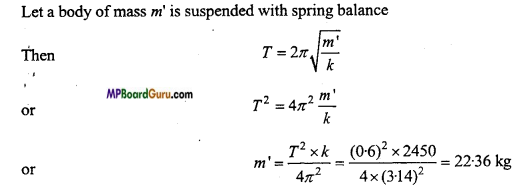Weight of the body
W = m’g
= 22.36×9.8 = 219.17 N
≈ 219N.

Question 14.
A spring having spring constant 1200 Nm’ is mounted on a horizontal table as shown in fig. A mass of 3 kg is attached to the free end of the spring. The mass is then pulled side ways to a distance of 2.0 cm and released. (NCERT)
Determine
(j) The frequency of oscillations.
(ii) Maximum acceleration of the mass and
(iii) The maximum speed of the mass.Solution:
Given:k=1200Nm-1,m= 3kg, amplitude a =2 cm =2 × 102m
(i) Frequency υ =$$\frac{1}{2 \pi}$$ $$\sqrt{\frac{k}{m}}$$ = $$\frac{1}{2 \times 3 \cdot 14} \sqrt{\frac{1200}{3}}$$
= $$\frac{1}{6 \cdot 28}$$ ×$$\sqrt{400}$$ =  \frac{20}{6 \cdot 28}
= 3.18 s-1 ≈3.2 -1

(ii) α = -ω 2 y
∴α max = ω 2a = $$\frac{k}{m}$$ .a = $$\frac{1200}{3}$$ ×2 ×10-2
= 8.0 ms -2

(iii) ∴ν max=ωa= $$\sqrt{\frac{k}{m}}$$ ×a =$$\sqrt{\frac{1200}{3}}$$ ×2 ×10-2
= 20 × 2 × 10-2 = 0.4 ms -1

Oscillations Class 11 Important Questions Objective Type

1. Multiple- choice questions:

Question 1.
Which of the following equation does not represent simple harmonic, motion:
(a) x = asin(ωt+δ)
(b) x = bcos(ωt+Φ)
(c) x = atan(ωt + Φ)
(d) x = asin(ωt cosωt.
Answer:
(c) x=atan(ωt + Φ)

Question 2.
Time-period and amplitude of a particle executing simple harmonic motion is Tand a. The minimum time taken to reach distance will be:
(a) T
(b) $$\frac{T}{4}$$
(c) $$\frac{T}{8}$$
(d) $$\frac{T}{16}$$
Answer:
(c) $$\frac{T}{8}$$

Question 3.
Time-period and amplitude of a particle executing simple harmonic motion is T and a. Its maximum velocity is :
(a) $$\frac{4 a}{T}$$
(b) $$\frac{4 a}{T}$$
(c ) $$2 \pi \sqrt{\frac{a}{T}}$$
(d) $$\frac{2 \pi a}{T}$$
Answer:
(d) $$\frac{2 \pi a}{T}$$Question 4.
Acceleration in S.H.M. is :
(a) Maximum at amplitude position
(b) Maximum at mean position
(c) Remain constant
(d) None of these.
Answer:
(a) Maximum at amplitude position

Question 5.
The ratio of acceleration to displacement of a particle executing S.H.M. is measurement of:
(a) Spring constant
(b) Angular acceleration
(c) (Angular acceleration)2
(d) Restoring force.
Answer:
(c) (Angular acceleration)2

Question 6.
When displacement is half of amplitude, then ratio of potential energy to the total energy is:
(a) $$\frac{1}{2}$$
(b) $$\frac{1}{4}$$
(c) 1
(d) $$\frac{1}{8}$$
Answer:
(b) $$\frac{1}{4}$$

Question 7.
What remain constant in S.H.M.:
(a) Restoring force
(b) Kinetic energy
(c) Potential energy
(d) Time-period.
Answer:
(d) Time-period.

Question 8.
Graph between ‘f and ‘F obtained as :
(a) Hyperbola
(b) Parabola
(c) Straight line
(d) None of these.
Answer:
(b) ParabolaQuestion 9.
The time-period of a seconds pendulum is :
(a) 1 second
(b) 2 second
(c) 3 second
(d) 4 second.
Answer:
(b) 2 second

Question 10.
Resonance is example of:
(a) Tuning fork
(b) Forced oscillation
(c) Free oscillation
(d) Damped oscillation.
Answer:
(b) Forced oscillation

2. Fill in the blanks:

1. A periodic motion of constant amplitude and same frequency is called ………… .
Answer:
Simple harmonic motion

2. In simple harmonic motion its total energy is ………. .
Answer:
Constant

3. The restoring force setup per unit extension in the spring is called ………… .
Answer:
Spring constant (Force constant)

4. When a body oscillates on both sides of its mean position in a straight line, then this bind of motion is called ………… .
Answer:
Simple harmonic motion

5. The maximum displacement of a body in oscillatory motion is called ………… .
Answer:
Amplitude

6. In the presence of damping forces, the amplitude of oscillations of a body ………… .
Answer:
Decreases

7. The time-period of second pendulum is ………… second.
Answer:
Two

8. The periodic time of simple pendulum does not depends upon
Answer:
Mass.3. Match the following:
I.

 Column ‘A’ Column ‘B’ 1. Maximum kinetic energy in S.H.M. (a) 2π$$\sqrt{\frac{\text { Displacement }}{\text { Acceleration }}}$$ 2.Maximum potential energy in S.H.M (b) Remain constant 3. Total energy of S.H.M. (c) At mean position 4. Acceleration of S.H.M. (d) At maximum displacement 5. Time-period of S.H.M. (e) Directly proportional to displacement.

Answer:
1. (c) At mean position
2. (d) At maximum displacement
3. (b) Remain constant
4. (e) Directly proportional to displacement.
5.  (a) 2π$$\sqrt{\frac{\text { Displacement }}{\text { Acceleration }}}$$.

II.

 Column ‘A’ Column ‘B’ 1. Motion of moon (a) Forced oscillation 2. Resonance (b) Conservation of energy and momentum 3. S.H.M. (c) Periodic motion 4. Mechanical waves (d) Main frequency 5. Minimum frequency of vibration (e) S.H.M.

Answer:
1. (c) Periodic motion
2. (a) Forced oscillation
3. (e) S.H.M.
4. (b) Conservation of energy and momentum
5. ((d) Main frequency.4. Write true or false:

1. Every S.H.M. is a periodic motion.
Answer:
True

2. Every periodic motion is S.H.M.
Answer:
False

3. In S.H.M. total energy is directly proportional to square of amplitude.
Answer:
True

4. Acceleration is always zero for a particle executing S.H.M.
Answer:
False

5. Time-period of simple pendulum is directly proportion to square root of its length.
Answer:
True

6. Time-period of simple pendulum is inversely proportional to the square root of acceleration due to gravity.
Answer:
True

7. Time-period of simple pendulum depends on mass, material and size of the pendulum.
Answer:
False

8. In S.H.M., velocity is maximum when acceleration is minimum.
Answer:
True

9. The motion of moon around the earth is S.H.M.
Answer:
False

10. Time-period of hard spring is less than soft spring.
Answer:
True

11. Time-period of a simple pendulum cannot be one day.
Answer:
True

MP Board Class 11th Physics Important Questions